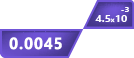# Scientific Notation Converter

x 10^

## Other Calculators

The above-displayed tool is known as the scientific notation converter. The main purpose of this tool is to convert scientific notation to other types of numbers.

This means it converts scientific notations whether to or from other numbers. It can be used to transform scientific notations into:

• E-notation
• Engineering notation
• Decimal system

You can copy any of the converted numbers by clicking on the copy icon.

## How to use Scientific Notation Converter?

To use this tool, follow the instructions below;

1. Enter the decimal value and the exponential part.
2. Click “calculate”

That’s it. Isn’t it easy? If you want to find the scientific notation of a whole number then after entering the number, input the power of base ten as zero.

## What is Scientific Notation?

To put it simply, Scientific notation is an easy way of writing difficult numbers. Difficult numbers refer to very very small or extremely large numbers. Such as 0.000000000000123.

Since such numbers are hard to read and while writing, there is a great risk of error, these numbers are converted into a much easier form known as scientific notation.

For example, if 0.000000000000123 is converted into scientific notation then it is 1.23 x 10-13. By looking at this number you can make some observations.

Once you get your answer, you can use the sig fig calculator to count how many significant figures in the answer.

First, the decimal is after the first non-zero digit. Then you can see that the digits are counted and the number is raised as the power of 10.

Powers can be positive or negative depending on the type of the number.

Example:

Convert the number 8900000 into scientific notation.

Solution:

Step 1: Place the decimal point.

= 8.900000

Step 2: Count the numbers.

After the decimal point, there are 6 numbers.

Step 3: Raise this number as the power of base 10.

= 8.9 x 106

Fun fact: One million in scientific notation is 1 x 106. Try converting one billion in scientific notation.

## How to convert from scientific notation?

Continue reading to learn how to convert scientific notation to other formats.

Into decimals:

Expand the base 10. And write the full number. For example, 1 x 106 in a decimal system is 1000000.

Into E-notation:

The only difference between e-notation and scientific notation is that in e-notation the base 10 is replaced with e. For example, the number is written as 1e6

Usually, the power is written as integers to distinguish. Like 1e+6. If the power was negative the symbol would have been minus (-).

Into engineering notation:

It is also similar to the previously mentioned formats. The difference here is one condition namely the power of 10 must be multiple of 3.

For instance, if you want to convert 1 x 107 into engineering notation, there are two ways to do it. Increase the power to 9 or decrease it to 6 i.e 0.01 x 109 and 10 x 106 respectively.